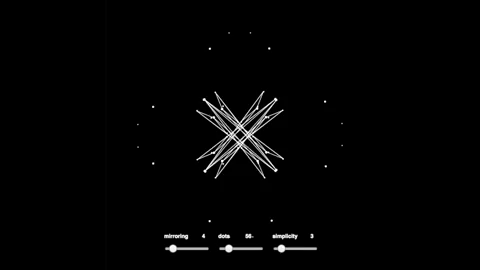top of page

# ICM | Week 3 | KaleidoscopeThis week is the pair assignment, and the requirement is to create repetition with variation.

After brainstorming with my buddy, Ryan Grippi, we decided on making a kaleidoscope, because it repeats itself, has variations, and it looks pretty.

We divided the assignment like so:

• I was to work on drawing the kaleidoscope algorithmically.

• Ryan to work on the user interface for tweaking the kaleidoscope.

To compile, Ryan would use the variables I created in his interface functions.

I started with trying to understand how kaleidoscope works. I knew there are mirrors involved, and the patterns are created by reflecting beads inside the kaleidoscope over and over again.My initial plan on how to code kaleidoscope up.

Initially, this was how my brain deconstructed drawing a kaleidoscope

• Translate the axes to the middle of the canvas

• Draw a right-angled triangle

• Rotate the axes by 45 degrees and repeat 7 times

• Generate random things inside the triangle, do the same

I also stumbled upon this when I googled "how to make kaleidoscope with code".How pretty

But my brain couldn't figure out how to mask the dots and lines so that they are only visible within their triangle region. There is a function called mask(), but that's for preloaded graphic asset.

Nonetheless, I tried coding the triangular sections.And I failed. Turned out I have to "mirror" the triangles as I recursively draw them, but the logic is a bit tricky since sometimes it should be reflected by y-axis, and sometimes x-axis. I could hardcode the triangles, but that would defeat the purpose because the recursive logic is meant to be applied to the dots and lines as well.

So I tried drawing 4 sections instead of 8 first.And then drawing the triangles again but in the opposite direction.And it kinda works (?). But the "mirroring" effect is not there at all :(

So I gave up, and just went ahead with adding the dots and lines to the sketch. Also, I deleted the triangles because it was pointless if I don't need the masking.

And voila!The details of how I coded this can be read from the source code, but basically the logic was:

• Draw dots with ellipse() function twice. One set to rotate clockwise, the other counter-clockwise

• Rotation is achieved through changing the dots' x & y coordinates using the formula "radius * cos / sin (rotation angle)" – rotation angle is incremented/decremented overtime.

• Lines are drawn using selected dots coordinates. This is achieved via a conditional statement.

• Draw the dots and lines recursively in other parts of the canvas, with the centre of the canvas as the centre point. Rotate() function is used to position the dots and lines. Rotation angle is determined via a variable.

I also prepared some variables to be used in Ryan's interface functions:

1. mirror: denotes the number of circular sections – basically how many times the dots and lines are "mirrored" in the canvas. Works weirdly when it's an odd number.

2. dAmt: the number of dots in the canvas

3. simplicity: the variable in the conditional statement responsible for drawing the lines. I use a % operator – it kinda behaves erratically sometimes, but mostly still within expected behaviour.

Source code of the final product can be found here!

See All
No tags yet.
bottom of page